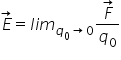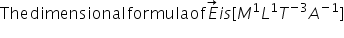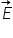# Electric Field

Electric field: The electric field at a point is defined as the electrostatic force per unit test charge acting on a vanishingly small positive test charge placed at that point. HenceThe electric field is a vector quantity whose direction is same as that of the force F exerted on a positive test charge.

In SI unit is Newton per coulomb. It is equivalent to volt per meter.Physical significance of electric field:

The force experienced by the test charge q0 is different at different points. Soalso varies from points to point.

By knowing electric field at any pint, we can determine the force on a charge placed at that point.

Electrostatic force = charge*electric field

Thus an electric field plays an intermediary role in the forces between two charges.

Charge electric field ↔ charge

Related Keywords